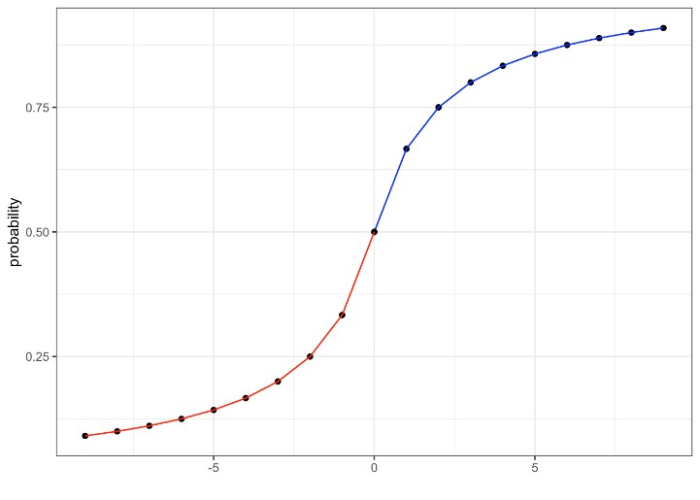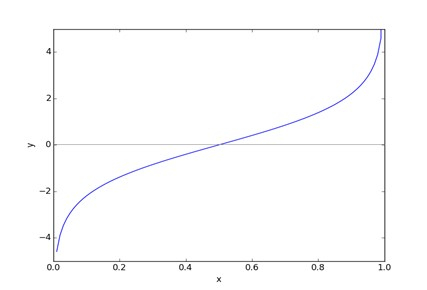# Role of Log Odds in Logistic Regression

## Introduction

Logistic Regression is a statistical method to predict a dependent data variable based on the relationship between one or more independent variables. It makes use of log odds and with the help of a logistic function, it predicts the probability of an event occurring. It is a classification method.

## What are Log Odds and Why are they Useful for Logistic Regression?

Logistic regression is used to predict binary outcomes. For example, in an election, whether a candidate will win or not, whether SMS is spam or ham, etc.

Odds are the ratio of the probability of success to failure. It is given as

$$\mathrm{Odds \:=\: p\: / \:1- p}$$

where p = odds of success, 1 – p = odds of failure

log of odds will be given by

$\mathrm{Log \:of\: odds\: = \:log \:(p\: /\: 1 - p)}$                                                                           (1)The above equation (1) is the logit function represented asFitting this equation to a line, we get

$\mathrm{log\frac{p}{1-p}\:=\:\beta_0x\:+\:\beta_1x}$        [ logistic regression equation]           (2)

$\mathrm{p\:=\:\frac{e^{\beta_0x\:+\:\beta_1x}}{1\:+\:e^{\beta_0x\:+\:\beta_1x}}}$                       (3)

odds of failure can be written as

$\mathrm{1-p\:=\:\frac{1}{1\:+\:e^{\beta_0x\:+\:\beta_1x}}}$                            (4)

The log odds ratio can be written as

$\mathrm{p/1-p\:=\:\frac{1}{1\:+\:e^{\beta_0x\:+\:\beta_1x}}\:=\:e^{\beta_0x\:+\:\beta_1x}}$                 (5)

which is the equation of logistic regression.

## Odds Ratio Example in Python

Let us take the following data.

country1

country2

cheetah

8

2

lion

1

5

### Example

from scipy.stats import fisher_exact as fe
odds_ratio, p_value = fe([[8, 2], [1, 5]])
print(odds_ratio)


### Output

20.0


• Log odds convert the Logistic Regression which is a probability-based model to a Likelihood–based model.

• Logistic Regression parameters are determined by Maximum Likelihood Estimation (MLE)

• They are very useful in solving problems of win or lose, fraud or non-fraud, span not span-like scenarios.

## Conclusion

Log odds are used in Logistic Regression when we want a non binary result. Log odds make the Logistic regression work both for regression and classification model

Updated on: 30-Dec-2022

3K+ Views